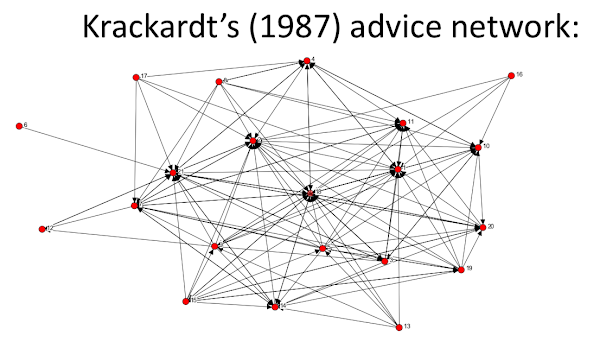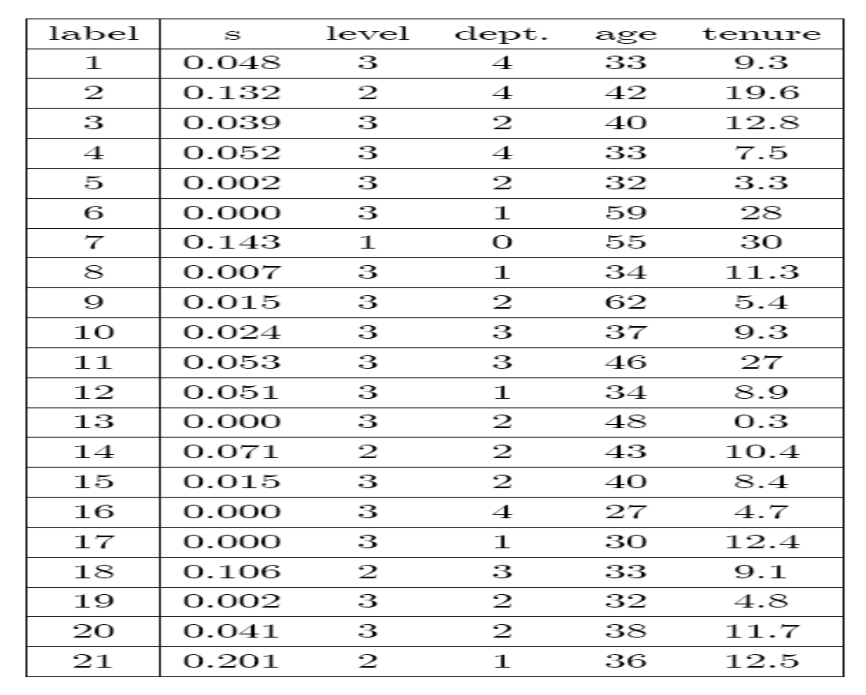# Stubborn Agents¶

• An agent who places high weight on self will maintain belief while others converge to that agent's belief

• Groups that are highly introspective will have substantial influence

# Another Example: Influence¶

• Suppose equally weight connections

• Suppose also that $T_{ij}>0$ if and only if $T_{ji} \ge 0$

• Suppose that, that actually what people did was equally weight their, their connections, and let's also suppose that you know, talking the that we think of this as friendships, so they're mutual. So, Tij is, is greater than 0 if and only if Tji is, is equal to 0. So, if I listen to you, you listen to me.
• $d_i$ is i's out degree

• So, $T_{ij} = 1/d_i$ for each i and j that i has a (directed) link to: so weight friends and weight them all equally

• So we have conversations and I put equal weight on all my connections. so if you think then of a situation where di is i's out degree. then Tij is going to be 1 over di, for each i and j. So, everybody that I am talking to, I put equal weight on, so however many friends I have, I give one if I have ten friends, I give 1 10th weight to each one of them. So let's think of that as a very simple version of this model.

# Example: Influence¶

Let $D = \sum_k d_k$

Claim: $s_i = d_i/D$ for each i

• Recall s is unit eigenvector: $s_i = \sum_j T_{ji} s_j$

• Verify that $s_i = \sum_j T_{ji} s_j$

• $s_i = \sum_j T_{ji} s_j = \sum_{j:T_{ij}>0}(1/d_j)d_j/D = d_i/D$

• $\sum_{j:T_{ij}>0}$: this sum is the sum over all the people that i listen to, is proprotional to the number of individuals that i is listening to
• So in a situation where you put equal weight on all your friends and listening is reciprocal, you get back degree centrality, so eigenvector centrality and degree centrality actually coincide.Now, it's a directed network, so certain people could actually listen to others without them listening back. So, this would be a directed network not necessarily back and forth. And there's some individuals who actually aren't connected. So, some individuals are not getting listened to at all and their influence is going to turn out to be zero.

In this situation, so it's not a strongly connected network. Nonetheless, the S is still going to work as the the right answer to this. so if you go through and figure out what the S is. So if you solve for the S of this, there are some individuals, for instance 6 who didn't get listened to and 13 and so forth, end up with 16 and 17, so some people end up with no influence, nobody listens to them and comes to them for advice. but basically we end up with the advice levels varying from 0 up to let's see, we've got a 0.2 here. so we end up with different levels of influence.

And what the other columns in this table represent is sort of, these are levels of the individuals in a hierarchy in this company. so level one, this is the CEO, the head of the company. Level two, we've got people at the second highest management level. Then level three, is the third-highest level. And what we see is actually there's some people that are more influential in terms of this network than the, the individual at the top, actually one of the people at level two has a higher influence vector than the person at the top.

And we can begin to look at different people, different people in, at, at level three have different levels of influence. And you know, you can look at that by the different department, their age, their tenure, and so forth. And this information is going to be complimentary to some of those things that doesn't just necessarily correlate with how old they are or how long they have been in the company or which department they're in. These numbers are telling you something different about the, the relative influence of these individuals have.

so what that does is it just shows, you know, one example of where you can begin to take this DeGroot model. It gives us a foundation for looking at this particular left-hand side unit eigenvector as a, a measure of influence. And you know, if you ran the DeGroot process on this particular network. And had people updating over time in their beliefs, that would tell you what their beliefs would eventually converge to. And this was done under the assumption that so we don't know exactly what these weights are. That people put equal weights on each one of their friends that they set. So person 17, for instance has you know five out arrows. So they put 1 5th weight on each one of those.

# Influence¶

• Provides foundation for eigenvector-based centrality or power measures

• Saw relation of eigenvector to walks - $g^t$ gives measures of all walks of length t...

In :
b = [0.2 0.4 0.4; 0.8 0.2 0;0.8 0 0.2]

Out:
3×3 Array{Float64,2}:
0.2  0.4  0.4
0.8  0.2  0.0
0.8  0.0  0.2
In :
for i = 1:30000
tmp = b^i
if tmp[1,:] == tmp[2,:]
return i
end
end

Out:
77
In :
b^77

Out:
3×3 Array{Float64,2}:
0.5  0.25  0.25
0.5  0.25  0.25
0.5  0.25  0.25